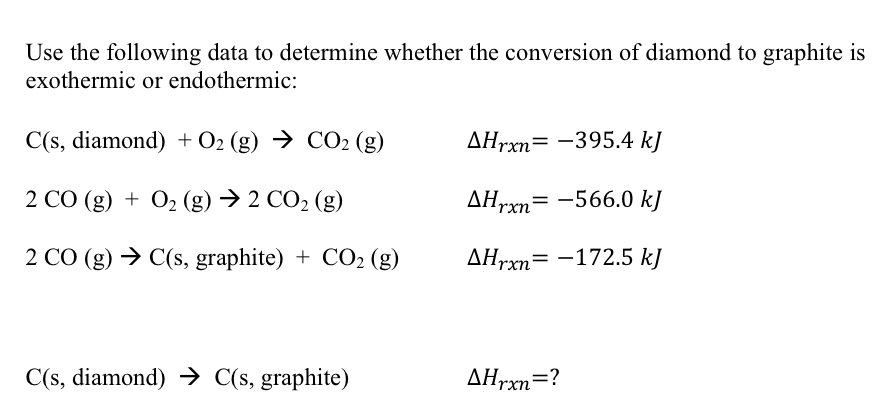Home / Answered Questions / Other / use-the-following-data-to-determine-whether-the-conversion-of-diamond-to-graphite-is-exothermic-or-e-aw948

# (Solved): Use The Following Data To Determine Whether The Conversion Of Diamond To Graphite Is Exothermic Or E...Use the following data to determine whether the conversion of diamond to graphite is exothermic or endothermic: C(s, diamond) + O2(g) â†’ CO2 (g) AHrxn= -395.4 k] 2 CO (g) + O2 (g) â†’ 2 CO2 (g) AHrxn= -566.0 kJ 2 CO (g) â†’ C(s, graphite) + CO2 (g) Hrxn= -172.5 kJ C(s, diamond) â†’ C(s, graphite) AHrxn=?

We have an Answer from Expert